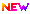High Impact Factor : 4.396| Submit Manuscript Online|

## ON Fuzzy Linear Programming Technique Application

### Author(s):

Dr. R. S. Chanda , University of Calcutta,

### Keywords:

Fuzzy set theory, Crisp linear Programming, Fuzzy linear Programming, Optimization, Membership function.

### Abstract

A solution approach based on fuzzy linear programming is proposed and applied to optimal machine scheduling problem. In this solution approach errors in the demand of various products during the next production period are considered to be fuzzy in nature. In conventional linear programming approach it is assumed that there is no error in the expected demand of various products. A fuzzy linear programming approach is proposed to obtain an optimal solution under fuzzy conditions. In the proposed method expected demand of products & profit are expressed by fuzzy set notations. The proposed fuzzy linear programming formulation is then transformed to an equivalent conventional linear programming problem and solutions obtained by solving this transformed linear programming problem. For illustration purpose the proposed method is applied to a profit maximization related machine scheduling problem.

### Other Details

Paper ID: IJSRDV1I4034
Published in: Volume : 1, Issue : 4
Publication Date: 01/07/2013
Page(s): 953-957